# Research of the hydraulic resistance of the inertial scrubber

Исследование гидравлического сопротивления инерционного скруббера
Цитировать:
Research of the hydraulic resistance of the inertial scrubber // Universum: технические науки : электрон. научн. журн. Tojiev R. [и др.]. 2021. 7(88). URL: https://7universum.com/ru/tech/archive/item/12084 (дата обращения: 06.07.2022).
Прочитать статью:
DOI - 10.32743/UniTech.2021.88.7.12084

ABSTRACT

The article proposes a design scheme of an inertial scrubber for wet industrial secondary gas cleaning. The method of determining the hydraulic resistance of the device is developed and the equation for determining the coefficient of resistance of the device is given. In experimental studies, gas velocity, fluid flow, and injector hole diameter were selected as variables. For different values of the factors, the coefficient of resistance and hydraulic resistance of the working bodies of the device was determined. The results of the experiment were obtained from the empirical formulas that adequately represent the process using the method of mathematical planning.

АННОТАЦИЯ

В статье предложена конструктивная схема инерционного скруббера, очищающего промышленные вторичные газы влажным способом. Разработана методика определения гидравлического сопротивления устройства и приведено уравнение для определения коэффициента сопротивления устройства. В экспериментальных исследованиях в качестве переменных факторов выбираются скорость газа, расход жидкости и диаметр отверстия форсунки. При различных значениях факторов определяются коэффициенты сопротивления и гидравлическое сопротивление рабочих органов устройства. По результатам эксперимента были получены эмпирические формулы, адекватно выражающие процесс при помощи математического метода планирования.

Keywords: hydraulic resistance, fluid flow, fluid layer, injector, mass transfer, correction factor.

Ключевые слова: гидравлическое сопротивление, поток жидкости, слой жидкости, инжектор, массообмен, поправочный коэффициент.

Introduction. The devices used in the purification of industrial secondary gases are selected based on the physical and chemical composition of the purified gas, its average median size and the different mechanisms of the gas. There are dry and wet methods of secondary gas purification in the industry, these types of devices are considered to have low hydraulic resistance and high operating efficiency. But the level of dry cleaning is much lower than the level of manual cleaning. Therefore, today in the world there is a tendency to use the wet method in the purification of industrial dust and secondary gases. This method also has its disadvantages, for example, the energy consumption for the cleaning is higher than the dry method, which has to recycle dust and gases absorbed into the liquid medium. In addition, the efficiency of the devices does not always meet the requirements of the existing environmental standards on the level of maximum allowable concentration (MAC) of harmful substances released into the atmosphere. This is mainly due to external influences loaded on the device and the high addition of dust and secondary gases to the gas flow. New effective methods or external energy exposures will need to be applied to increase the likelihood of dust and secondary gases colliding with liquid droplets . For example, a liquid used to purify gases at high temperatures evaporates. But it is not used to purify gases from steam. If ways and means of using this steam are created, the energy spent on cleaning can be reduced by 50%.

In addition, most devices currently in use come into contact with the fluid at a specific contact element. This, in turn, requires setting a finite value of the gas velocity and the amount of particles in the stream. Therefore, the mass transfer processes slow down or complete mass transfer becomes difficult. In this regard, it is more efficient to identify and apply the method of inertial transfer of gases directly to the internal environment of the liquid.

Currently, a lot of research is being done in this area [2-5]. Among them are J.H.Walther, H.C.Lu, C.J.Tsai, K.Morris, C.Cursley, E.Rothwell, Yu.G.Nechaev, A.F.Sorokopud, D.V.Khokhlov, I.M.Kavashnin, E.S. Nechaeva, K.S. Polotnikov's research work deserves special recognition.

These types of devices are highly efficient and have a gas cleaning efficiency of up to 99%. But the energy savings of the devices are not so high. Therefore, when choosing a particular structure, it is necessary to take into account a set of technical and economic characteristics. In addition, given the similarity of the characteristics of the devices in research work, the issues of determining the optimal performance and reducing energy consumption at work are relevant.

The object of research. Based on the above, a new design scheme of the industrial secondary gas cleaning inertial scrubber and a laboratory version for experimental research were developed (Figures 1 and 2).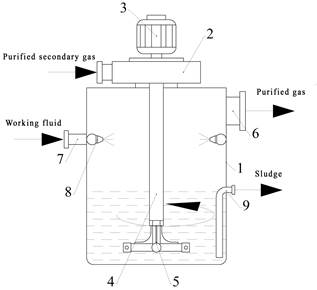Figure 1. Constructive scheme of the inertial scrubber:

1 - body; 2 - fan; 3 - electric motor; 4 - guide tube; 5 - rotating injector; 6 - purified gas pipe; 7 - fluid pipe; 8 - nozzle; 9 - sludge pipe.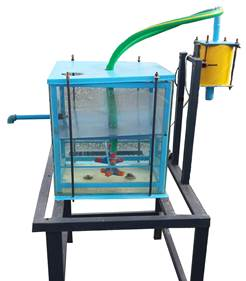Figure 2. General view of the inertial scrubber laboratory sample

The device consists of a rotating syringe and a secondary gas supply pipe and fan, a cylindrical vertical body equipped with a liquid spray nozzle, a collecting bath and a sludge pipe, and a pipe for the release of purified gas into the atmosphere. a syringe (four rotating 90 ° injectors are mounted on the rotating syringe and it is attached to the guide tube with a seal and a fastening ring. The nozzle holes are placed parallel to the liquid.) is immersed in a bath in which the working fluid is collected. As the purified gas passes to the syringe, the injectors rotate in the liquid medium according to the difference between the gas pressures in the guide tube and the syringe. The gas to be cleaned is discharged through the nozzle holes and is in contact with the working fluid. The gas is purified during its movement in the liquid medium. The purified gas is released into the atmosphere through a tube. The main advantage of the device over existing scrubbers is that, firstly, the use of working fluid increases due to the rotation of its injectors in the liquid medium, and secondly, the rotational motion of the syringe provides a curvilinear movement of gas flow in the liquid medium. This in turn increases the mass transfer coefficient.

However, insufficient research has been conducted to substantiate the effect of operating parameters of inertial devices, including hydraulic resistance, on cleaning efficiency. Therefore, this work is aimed at substantiating the optimal parameters of the inertial device.

Research results. To keep the viscosity and level of the working fluid in the device and to re-purify the secondary gases emitted by the untreated air in the working body, the nozzle S32-412 (hole diameter dn = 3 mm, 4 pieces according to the diameter of the device body) was installed. α was chosen to be 90 °C accordingly.

Figure 3 shows the general view of the nozzle), centrifugal pump (Qmax = 40 l/min; Ndv = 0.37 kW; hmax = 38 m; V = 220 V; nrpm = 3000 rpm/min according to GOST-2757030-91), rotometer (RS- 5; scale indicators in the range 0 ÷ 100; according to GOST-13045-81) and a beaker for weighing (full volume 3.2 l) was selected. Fluid flow and velocity were determined using the volumetric method for the diameter of the nozzle hole dn = 3 mm. To do this, the filling time of the beaker was determined according to the readings of the rotometer 0 ÷ 90.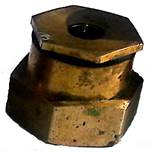Figure 3. View of nozzle

In the experimental determination of fluid flow, each experiment was repeated 5 times, and the square dimensions of each point and the resulting errors were determined. When the rotometer scale readings changed to 0 ÷ 90, the fluid flow rate changed to Q = 0.068 ÷ 0.360 m3/h. In each indicator, the flow change increased by 0.044 m3/h step.

Local resistances to the working fluid are affected in the fluid pipe and nozzle. The magnitude of the coefficient of friction in turbulent flow depends on the mode and the roughness of the pipe. If the working fluid supplied to the device passes through the pump and the liquid pipe and the novelty of the selected pipe is taken into account, then it is possible to assume that the local resistance coefficient at a distance to the nozzle is 1.2 [5, 6, 7].

We use the research results of B.A. Alimatov and I.T. Karimov to determine the coefficient of resistance of the nozzle hole to the liquid flow. According to him, the thickness of the nozzle hole was determined on the graph of the ratio of the hole to the diameter of the hole. If the diameter of the nozzle hole selected for the device is 3 mm and the hole thickness is 1.2 mm, then the coefficient of resistance in the nozzle is 2.5. in which case the total resistance coefficient is 3.7. Experiments were carried out in accordance with RD 34.20.519-97 “Testing of hydraulic resistance of pipelines. Machines and apparatus for measuring gas consumption and pressure. Program and methods of testing ” [8,9,10].

To determine the gas velocity, consumption and resistance coefficients of the working bodies of the device (Operational efficiency Qmax = 350 m3/h; electromotive force Ndv = 1.5 kW; rotation frequency n = 1800 rpm) of the centrifugal type, gas velocity ANEMOMETER VA06 -TROTEC (measuring range 1.1 ÷ 50 m/s, error coefficient 0.2%, gas velocity exceeding 50 m/s, error coefficient up to 5%) electronic meter, device for determining the pressure of dusty gas in the working bodies of the device JM- Electronic measuring device brand 510, the automatic adjuster (measuring line in the range of 0 ÷ 20 mm) is installed on the fan to control the gas velocity. In order to transfer the purified gas to the liquid medium, a four-stage injector (puller) with a hole diameter df = 2.3.4 mm and a thickness of 3.5 mm was used. Figure 4 shows an overview of the puller. Each experiment was repeated 5 times.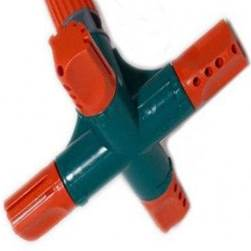Figure 4. General view of the puller

Based on the obtained experimental values, it was studied that the total resistance coefficient of the device depends on the above-mentioned factors. In this case, the equation for determining the coefficient of the total resistance of the moving gas flow in the device to the working bodies can be written as follows ;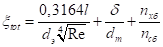(1)

Using Equation (1), we determine the coefficient of resistance of the pipe, syringe, and fluid medium that directs the working bodies of the device. The study to determine the coefficient of resistance was conducted in three stages. In the first stage, the resistance coefficient in the guide pipe was investigated. A lot of scientific research has been done to determine the local resistance coefficient in a guide pipe. For example, Darsi, Idelchik, and Konokov found that the coefficient of friction depends on the plane of the inner surface of the pipe when the flow regime of the gas moving in the pipe is turbulent. Studies have shown that if the pipe is new, the local resistance coefficient is 1. Taking into account the turbulent transition of the gas supplied to the device under study and the novelty of the selected pipe, it can be assumed that the coefficient of local resistance at the distance to the syringe is equal to 1 for different values of the Variables in the experiments [5,6].

In the second stage, the resistance coefficient in the syringe injector was studied. An effective method is to determine the coefficient of resistance in the syringe injector using the research results of B.A. Alimatov and I.T. Karimov .

An overview of the puller is shown in Figure 4 and the experimental results are shown in Figure 5. Experiments were carried out in accordance with RD 34.20.519-97 “Testing of hydraulic resistance of pipelines. Machines and apparatus for measuring gas consumption and pressure. Program and methods of testing ” .Figure 5. The dependence of the coefficient of resistance of the nozzle (ξш) on the diameter ratio (δ/dш) of the hole thickness.

From the data given in Figure 5, it can be seen that as the diameter of the injector hole increases, the area of exposure to the secondary gas decreases and the efficiency of the transition of the gas to the liquid medium increases. This, in turn, leads to an increase in the volume of gas bubbles dispersed in the liquid medium and a decrease in the mass transfer coefficient (Figure 6).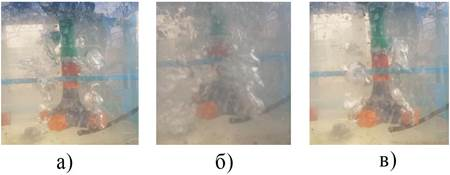Figure 6. The appearance of gas bubbles formed in the working fluid environment:

a-nozzle hole diameter 2mm; b-nozzle hole diameter 3mm; v-nozzle hole diameter 4mm.

The following empirical formula was obtained using the least-squares method for the dependencies shown in Figure 5 .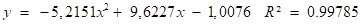(2)

In the third stage, the coefficient of resistance of the working fluid to the gas flow was determined experimentally. Determining the coefficient of resistance of a working fluid to a gas flow is complex and requires various deviations. Therefore, using Equation (1), we determine the coefficient of resistance by the ratio of the number of revolutions nхб to the number of revolutions under the influence of the working fluid pressure nсб under the influence of the natural air pressure of the synergist. Table 1 shows the differences in the number of cyclic rotations in the above two cases.

Table 1.

Differences in the number of cyclic cycles at different values of the variables.

 Speed control indicator 0 5 10 15 20 Puller hole diameter dc=2mm nхб 7 16 29 42 51 nсб 2.5 5.5 10 14 17 Puller hole diameter dc=3mm nхб 5 12 26 37 45 nсб 2 5 10.5 15 18 Puller hole diameter dc=4mm nхб 2 7 23 34 41 nсб 1 3.5 11 16 22

From the data given in Table 1, it can be seen that the resistance coefficient is 3 when dc=2mm; when dc = 3 mm the resistance coefficient is 2.5 and when dc = 4 mm the resistance coefficient is 2. The results of the general experiment showed that when dc = 2 mm, the total resistance coefficient of the device was 7.4 and the correction factor was 0.38; when dc = 3 mm the total resistance coefficient of the device is 6 and the correction coefficient is 0.62 and when dc = 4 mm the total resistance coefficient of the device is 4.6 and the correction coefficient is 0.87. The graph of the correction factor dependence on the resistance coefficient is shown in Figure 7.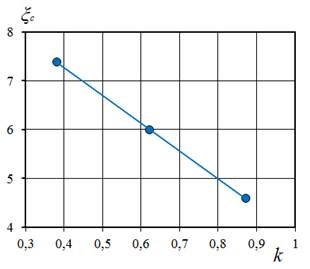Figure 7. The correction factor k varies with respect to the resistance coefficient ξ.

The following empirical formula was obtained using the least-squares method for the dependencies shown in Figure 7 .(3)

The hydraulic resistance of the device was investigated using the total resistance coefficients determined for different values of the variable factors.

The following parameters of the variables in determining the hydraulic resistance of the device, the gas velocity υг=7÷25.6 m/s, the intermediate step increased by an average of 4.5 m/s, the gas consumption Qг=150÷330 m3/h, the intermediate step by an average of 70 m3/h increased to, the diameter of the nozzle hole dt = 2; 3 and 4 mm, fluid flow Qliq=0,141; 0,168and 0.178 m3/h, the inert gas density was selected as ρг=1,29 kg/m3 (for air).

The temperature for the water and gas system was set at 20 °C ± 2, taking into account the influence of the external environment during the experiments. The results of the experiment were processed using an ECM and the dependence of hydraulic resistance on different values of variable factors was investigated in the absence of fluid supply to the device. Based on the results of the experiments, a comparison graph of the dependence of hydraulic resistance on the gas velocity was constructed. (Figures 8; 9 and 10).

The data in Figures 8, 9 and 10 show that the variable factors increased the gas velocity υг=7÷25.6 m/s with an intermediate step averaging 4.5 m/s, and the gas consumption Qг=150÷330 m3/h with an intermediate step averaging 70 m3/h was observed to increase from ΔPсб=400 Pa to ΔPсб=2959 Pa for dt = 4 mm. While the intermediate step averaged ΔPсб=400 Pa, it was observed that for dt = 3 mm, ΔPсб=252 Pa increased from ΔPсб=3381. The intermediate step averaged ΔPсб=450 Pa and was observed to increase from ΔPсб=316 Pa to ΔPсб=4227 Pa for dt = 2 mm. The intermediate step averaged ΔPсб=600 Pa.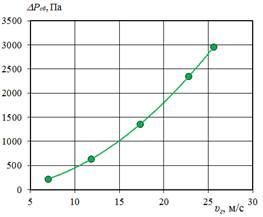Figure 8. The hydraulic resistance of the device depends on the gas velocity υг of ΔP.

when htot = 4.6 and ρг =1,29 kg/m3 - const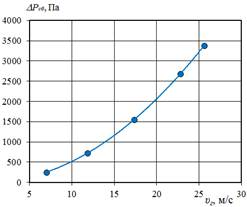Figure 9. The hydraulic resistance of the device depends on the gas velocity υг of ΔP.

when htot = 6 and ρг =1,29 kg/m3 – const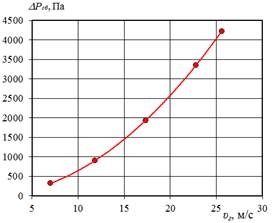Figure 10. The hydraulic resistance of the device depends on the gas velocity υг of ΔP.

when ξtot= 7,4 and ρг =1,29 kg/m3 – const

The following empirical formulas were obtained using the least-squares method for the graphical dependencies shown in Figures 8, 9 and 10 .

when ξtot = 4.6 and ρг = 1.29 kg/m3 – const;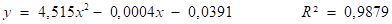(4)

when ξtot = 6 and ρг = 1.29 kg/m3 – const;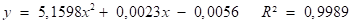(5)

when ξtot = 7.4 and ρг = 1.29 kg/m3 – const;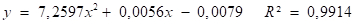(6)

Conclusion. From experiments to determine the hydraulic resistance, it can be concluded that the change in the coefficient of resistance in the device depending on different values of the nozzle diameter leads to an increase in hydraulic resistance and an improvement in cleaning efficiency. In addition, changes in the velocity and density of the gas supplied to the device also have a significant effect on the hydraulic resistance. As the hydraulic resistance increases, the cleaning efficiency improves, but the energy consumption for cleaning the dusty gas increases.

References:

1. Nurmuhamedov H.S., Temirov O.Sh., Turobjonov S.M., Yusupbekov N.R., Zokirov S.G., Tadjixujaev Z.A. Gas processing technology, processes and devices. – Tashkent: Sharq, – 2016. – 856 p.
2. Варваров В.В. Проблемы улавливания пылевидных фракций в тех-нологии сыпучих пищевых продуктов. – Известия вузов. Пищевая технология, – Кубан, 1988. – № 4. – С.27-35.
3. Швыдкий В. С., Ладыгичев М. Г. Очистка газов. Теплоэнергетик, Москва. – 2002. – 640 с.
4. Кобзарь И. Г. Процессы и аппараты защиты окружающей среды. Москва: “Драфа”, – 2008. – 239 с.
5. Исомидинов А.С. Разработка эффективных методов и устройств очистки пылевых газов химической промышленности: Дисс. … PhD. – Ташкент, 2020. – 118 с.
6. Исомидинов А. С. Исследование гидравлического сопротивления роторно-фильтрующего аппарата //Universum: технические науки. – 2019. – №. 10-1 (67).
7. Isomiddinov A. et al. Application of rotor-filter dusty gas cleaner in industry and identifying its efficiency //Austrian Journal of Technical and Natural Sciences. – 2019. – №. 9-10.
8. Архипов В.А., Березиков А.П. Основы теории инженерно-физического эксперимента. – Томск: Изд-во Томского политехнического университета, – 2008. – 206 с.
9. Бронштейн Б.И., Фишбейн Г.А. Гидродинамика, массо и теплообмен в дисперсных системах. – Ленинград: Химия, 1977. – 279 с.
10. Доспехов Б.А. Методика полевого опыта. – Москва: Колос, – 1978. –335 с.
11. A.O.Xoshimov, A.S.Isomidinov. Study of hydraulic resistance and cleaning efficiency of dust gas scrubber // International online scientific-practical conference on "Innovative ideas, developments in practice: problems and solutions": Andijan. – 2020. – 51 p.
12. Karimov IT, Alimatov BA, Analysis of experimental research in determining the flow rate of heavy liquids // Scientific and technical journal of Fergana Polytechnic Institute, – Fergana, 2019. – №4. – pp. 103–110.
13. Кобзарь А.И. Прикладная математическая статистика. Для инженеров и научных работников. – Москва: Физматлит, – 2006. – 816 с.
Информация об авторахDoctor of Technical Sciences, Professor, Fergana Polytechnic Institute, Republic of Uzbekistan, Fergana

д-р техн. наук, проф., Ферганский политехнический институт, Республика Узбекистан, г. ФерганаPhD, Associate Professor, Fergana Polytechnic Institute, Republic of Uzbekistan, Fergana

д-р техн. наук (PhD), доц. Ферганский политехнический институт, Республика Узбекистан, г. ФерганаDoctoral student, Fergana Polytechnic Institute, Republic of Uzbekistan, Fergana

докторант (PhD), Ферганский политехнический институт, Республика Узбекистан, г. ФерганаAssistant of Fergana Polytechnic Institute, Republic of Uzbekistan, Fergana

преподаватель, Ферганский политехнический институт, Республика Узбекистан, г. Фергана

Журнал зарегистрирован Федеральной службой по надзору в сфере связи, информационных технологий и массовых коммуникаций (Роскомнадзор), регистрационный номер ЭЛ №ФС77-54434 от 17.06.2013
Учредитель журнала - ООО «МЦНО»
Главный редактор - Ахметов Сайранбек Махсутович.
Top